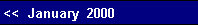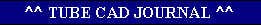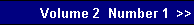We know what is required to make this amplifier naturally quiet: along with the music signal, no noise on the top tube's grid and all the negative power supply rail noise on the bottom tube's grid. The advantage a bipolar power supply holds over a mono-polar power supply, is that much of the noise on each rail is both equal amplitude and anti-phase with the other. Of course, this assumes an equal load for each rail voltage. This means that the midpoint between these rails is noise free, just as the knot in between the two rubber bands remains still while both ends are vigorously pull to and away from each other. (Actually, wherever we define ground to be will also define the reference point that against which both signal and noise will be measured. This point, obviously enough, has no signal and no noise relative to itself.)   As the inputs to the differential input stage are referenced to ground, the common cathode resistor remains fixed at one end and at its other end bounces up and down with the negative power supply rail noise. This varying voltage defines a varying current through the resistor, which, in turn, is relayed through the two tubes into the two plate resistors. The first triode's plate resistor is effectively grounded at the output of the amplifier. Thus, the noise induced current change develops an in-phase noise signal at the connection between plate resistor and plate, which is then transmitted to the bottom output tube's grid.    On the other hand, the second triode's plate resistor is effectively connected to the positive 100 volt rail of the power supply. Thus, the noise induced current change develops an in-phase noise signal that expands and contracts the voltage across the plate resistor to the same degree as the positive rail voltage expands and contracts, which effectively nulls at the plate and the top output tube's grid.   In order for this noise nulling technique to work requires that the plate resistors value equal twice that of the common cathode resistor.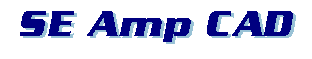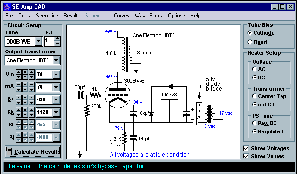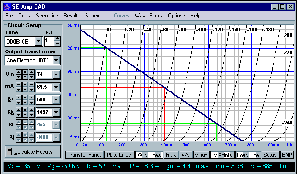pg. 12